Next: Gravity Waves Up: Dispersive Waves Previous: Waveguides

# Pulse Propagation in Two Dimensions

In Section 7.9, we saw that a wave can propagate through an inhomogeneous medium, without significant reflection, provided that the properties of the medium vary on lengthscales that are much longer than the wavelength of the wave. In this section, we shall determine the path taken by a localized wave pulse as it travels though such a medium. For the sake of simplicity, we shall restrict our analysis to two dimensions (by neglecting any variation in the-direction.)

The wavefunction of a two-dimensional wave propagating through a (slowly varying) inhomogeneous medium can be written in the general form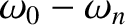(892)

Here,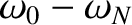is a relatively slowly varying function that determines the wave amplitude, whereas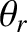is a relatively rapidly varying function that determines the wave phase. We can expandlocally as a Taylor series (see Appendix B) to give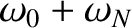(893)

Comparison of the previous two equations with the standard expression for the wavefunction of a plane wave (see Section 8.1),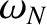(894)

which is assumed to hold locally at each point in space, reveals that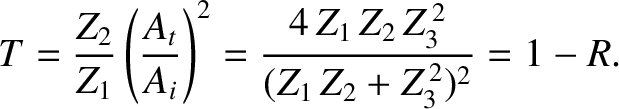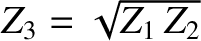(895)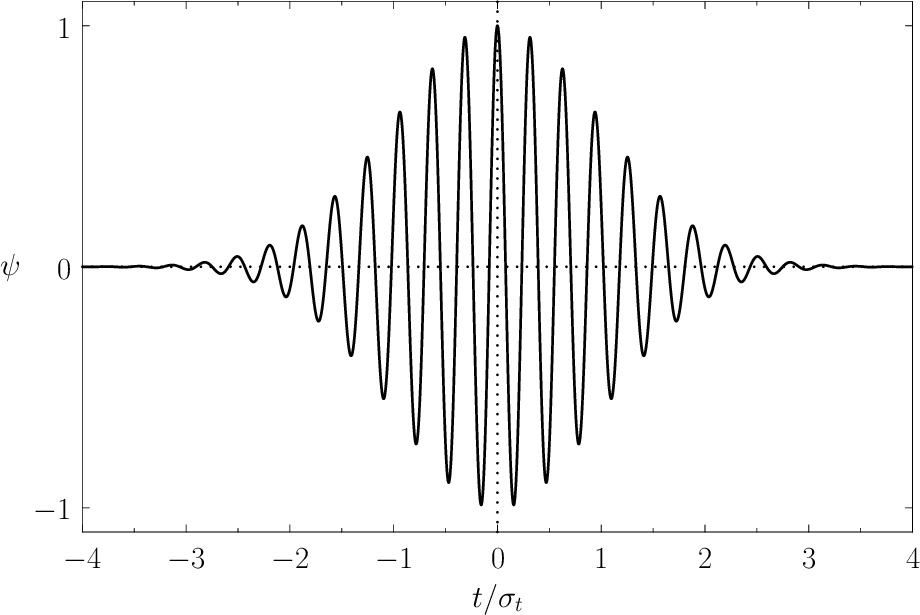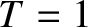(896)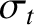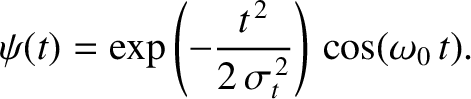(897)

where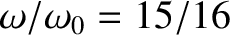is the wave angular frequency, and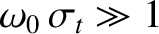, 0 ,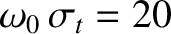the wavevector. (The generalization to three dimensions is straightforward.) The previous three equations also imply that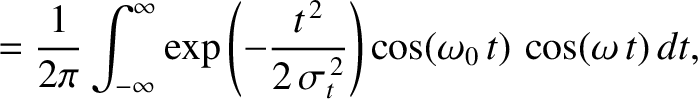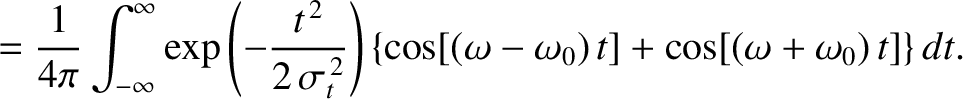(898)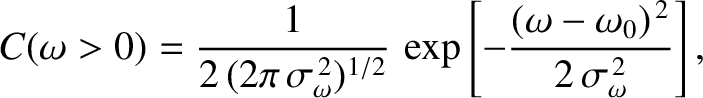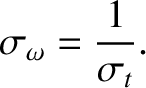(899)

As we saw in Section 10.1, a one-dimensional wave pulse propagating through a dispersive medium does so at the group velocity,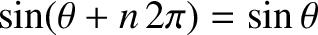, where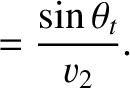is the dispersion relation. Thus, the equation of motion of the pulse is written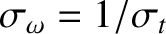(900)

In two dimensions, the dispersion relation (in a uniform medium) takes the form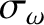. In this case, the expression for the group velocity generalizes to (Landau and Lifshitz 1959)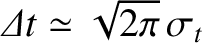(901)

(The generalization to three dimensions is straightforward.) Thus, in two dimensions, the equations of motion of a wave pulse are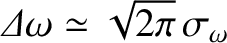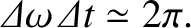(902)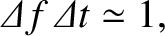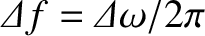(903)

It turns out that these equations also hold in a two-dimensional inhomogeneous medium: that is, a medium in which the dispersion relation is an explicit function of position: i.e.,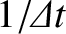. (Actually, this is only true as long as the dispersion relation varies on lengthscales that are much longer than the wavelength.) Thus, in a two-dimensional inhomogeneous medium, once we know the local dispersion relation,, we can trace the path of a wave pulse using the following four equations [cf., Equations (898)-(899) and (902)-(903)]:(904)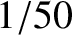(905)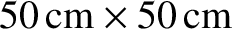(906)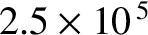(907)

(Incidentally,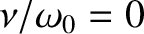,,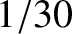, and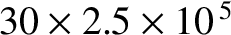are treated as independent variables in these equations.) It can be seen that the preceding equations are analogous to Hamilton's equations for two-dimensional motion in classical mechanics, with the wave vector playing the role of the momentum, and the function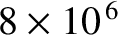playing the role of the Hamiltonian (Goldstein, Poole, and Safko 2002). (In fact, Hamilton's equations were first derived to determine the path of a light ray through an inhomogeneous medium, and only later applied to dynamical systems.)

Consider a radio wave pulse launched from the Earth's surface, and subsequently reflected by the ionosphere. Letmeasure horizontal distance, and letmeasure vertical height above the Earth's surface. The appropriate dispersion relation is (see Section 10.2)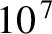(908)

where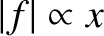is the velocity of light in vacuum, and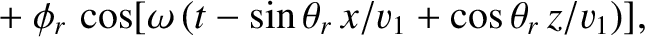the plasma frequency. This frequency is proportional to the square root of the number density of free electrons in the atmosphere, which we would generally expect to be a function of height only. In other words,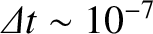. Equations (904)-(906) yield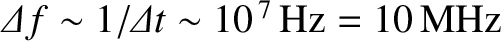(909)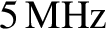(910)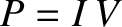(911)

It is helpful to rewrite the dispersion relation as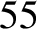(912)

where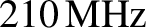is the refractive index.

Let us assume that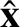at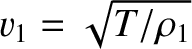, which is equivalent to the reasonable assumption that the atmosphere is non-ionized at ground level. It follows from Equation (911) that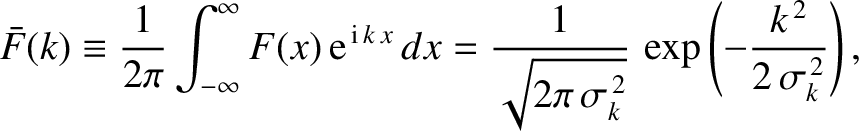(913)

where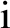is the sine of the angle of incidence of the pulse, with respect to the vertical axis, at ground level. The previous two equations can be combined to give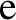(914)

According to Equation (910), the plus sign corresponds to the upward trajectory of the pulse, whereas the minus sign corresponds to the downward trajectory. Equations (909), (910), (913), and (914) give the following equations of motion of the pulse: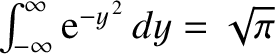(915)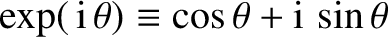(916)

It can be seen that the pulse attains its maximum altitude,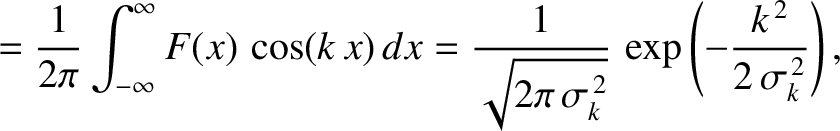, when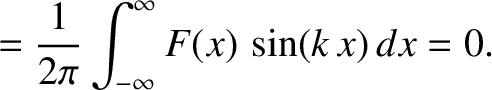. The total distance traveled by the pulse (i.e., the distance from its launch point to the point where it intersect's the Earth's surface again) is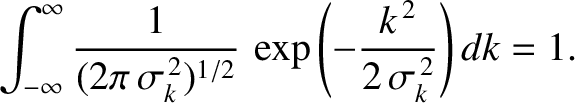(917)

According to Equations (909), (910), and (914), the equations of motion of the pulse can also be written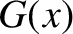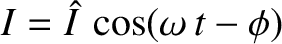(918)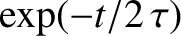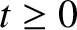(919)

It follows that the trajectory of the pulse is the same as that of a particle moving in the gravitational potential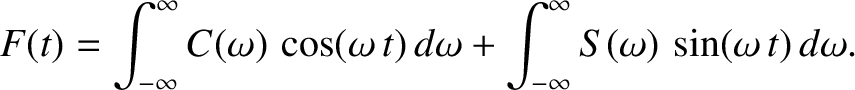. In particular, if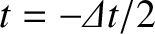decreases linearly with increasing altitude (as would be the case if the number density of free electrons increased linearly with) then the trajectory of the pulse is a parabola.Next: Gravity Waves Up: Dispersive Waves Previous: Waveguides
Richard Fitzpatrick 2013-04-08